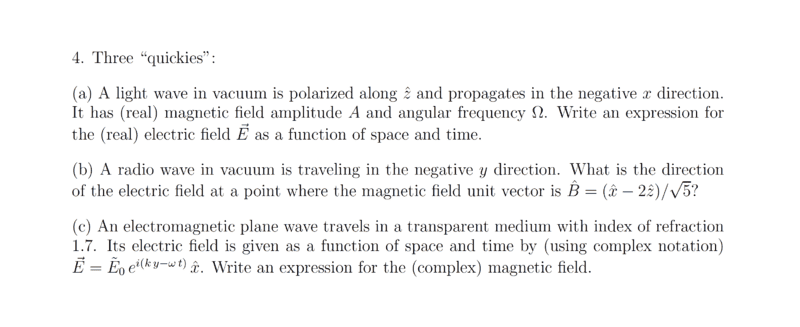# Determining Electric and Magnetic field given certain conditions

• guyvsdcsniper
In summary, the conversation touches upon the real part of a complex wave equation, with a negative factor indicating a left traveling wave, as well as the unit vector of a magnetic field and its relationship with the electric field. The conversation also discusses the use of equations and finding the correct answer through understanding and reading through a book.

#### guyvsdcsniper

Homework Statement
Refer to attached image
Relevant Equations
comlplex wave equation,I am unsure of my solutions and am looking for some guidance.

a.)The real part of the wave in complex notation can be written as ##\widetilde{A} = A^{i\delta}##. Writing the Complex Wave equation, we have ##\vec E(t) = \widetilde{A}e^{(-kz-\Omega t)} \hat x##. Therefore the real part is ##\vec E(t) =Ae^{(-kz-\Omega t+\delta)} \hat x##. The negative in front of kz indicates it is a left traveling wave.

b.) The unit vector of ##\hat B = \frac{(\hat x - 2\hat z)}{\sqrt{5}}##. I know that ##\hat E## must be perpendicular to ##\hat B##, so simply,
##\hat E = \frac{(\hat x + 2\hat z)}{\sqrt{5}}##

c.) I am not so sure about this problem. I know that ##\vec E = \widetilde{E}_oe^{i(ky-wt)}\hat x##
Griffiths states ##\widetilde{B}_o = \widetilde{E}_o/c## and ##v=c/n##.

So ##\vec B = \frac{c\widetilde{E}_o}{1.7}e^{i(ky-wt)}\hat z##

guyvsdcsniper said:
a.)The real part of the wave in complex notation can be written as ##\widetilde{A} = A^{i\delta}##. Writing the Complex Wave equation, we have ##\vec E(t) = \widetilde{A}e^{(-kz-\Omega t)} \hat x##. Therefore the real part is ##\vec E(t) =Ae^{(-kz-\Omega t+\delta)} \hat x##. The negative in front of kz indicates it is a left traveling wave.
The problem statement said the wave travels in the ##-x## direction. Your answer isn't sinusoidal. It decays with time. What is ##\delta##?

b.) The unit vector of ##\hat B = \frac{(\hat x - 2\hat z)}{\sqrt{5}}##. I know that ##\hat E## must be perpendicular to ##\hat B##, so simply,
##\hat E = \frac{(\hat x + 2\hat z)}{\sqrt{5}}##
It doesn't look like ##\hat E \cdot \hat B=0##.

•topsquark
vela said:
The problem statement said the wave travels in the ##-x## direction. Your answer isn't sinusoidal. It decays with time. What is ##\delta##?
You're right. I should have ##\vec E(t) =Acos{(-kz-\Omega t+\delta)} \hat x##
##\delta## is the phase
So I need to make ##\hat x## be ##\hat - x## as well as account for it is a wave traveling left by the ##-kz## in the ##cos##?

I assumed the ##-kz## in the ##cos## accounted for the negative direction.

guyvsdcsniper said:
I assumed the ##-kz## in the ##cos## accounted for the negative direction.
In the ##-z## direction, not the ##-x## direction.

Hint: The wave travels in the negative ##x## direction and the ##\vec{B}##-field (sic!) is polarized in ##z##-direction. So the complex ansatz for ##\vec{B}## is (using the HEP physicists' convention concerning the signs in the exponential)
$$\vec{B}=A \vec{e}_z \exp(-\mathrm{i} \Omega t-\mathrm{i} k x), \quad \Omega,k>0.$$
Now just use the source-free Maxwell equations to get the dispersion relation ##\Omega=\Omega(k)## and the ##\vec{E}##-field!

I figured it out. I had a big misunderstanding on the equations I was using but took the time to read through my book and was able to come to the correct answer. Thanks all for the help!

•vanhees71